Home > CCA2 > Chapter 10 > Lesson 10.2.2 > Problem10-121

10-121.
1. For the polynomial function f(x) = x3 − 5x2 + 11x − 15, which of the following are possible factors? Homework Help ✎

1. (x + 1)

2. (x − 2)

3. (x + 4)

4. (x − 3)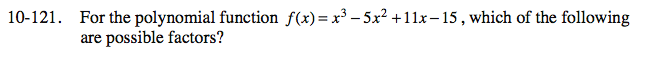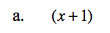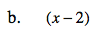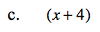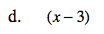The factored form of f(x) will be (x ± a)(x ± b)(x ± c).
What must be true about the product of a, b, and c?